# 基于飞桨实现高光谱影像和全色影像融合

2022/04/14 18:361.线性关系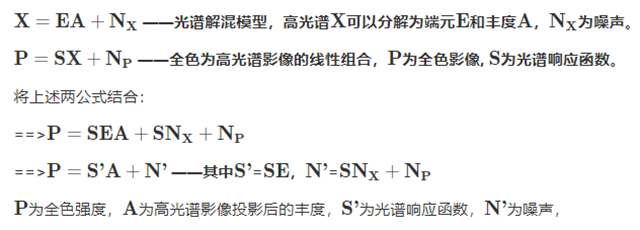2.非线性关系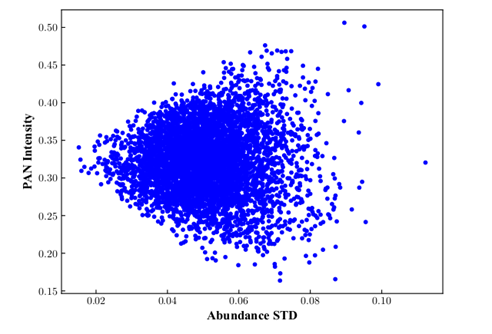1import numpy as np
2import matplotlib.pyplot as plt
4
5filename = '/home/aistudio/work/file/envi_plot3.txt'        # 选择的各种地物纯净光谱
7
8pixel_num = 5000
9abun = np.random.rand(pixel_num, curve_data.shape)
10abun_sum = np.sum(abun, axis=1)
11abun = abun / np.expand_dims(abun_sum, axis=1)
12
13intensity = np.dot(srf_pan, np.dot(curve_data[:, :], np.transpose(abun))[:srf_pan.shape, :]) / np.sum(srf_pan)
14x_var = np.std(abun, axis=1)    # 每个像元端元丰度的标准差
15
16plt.scatter(x_var, intensity, color='b', s=10)
17plt.xlabel('Abundance STD')
18plt.ylabel('PAN Intensity')
19plt.show()

3.细节注入子网络（PDIN）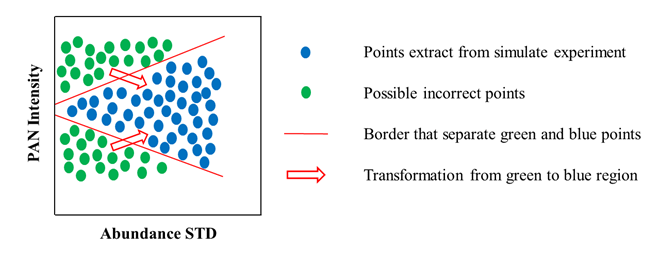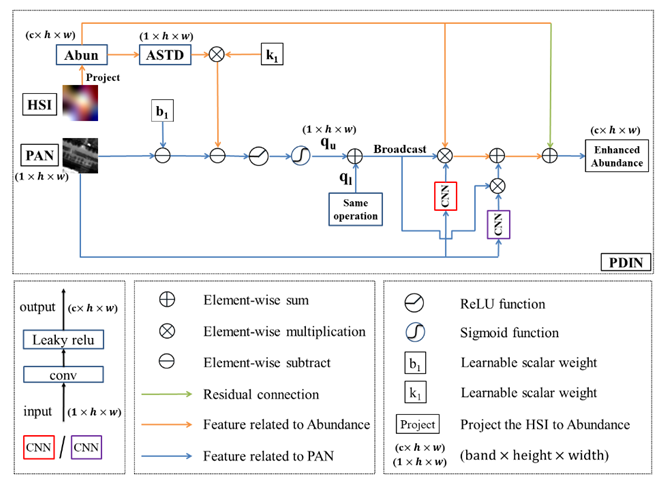1# 利用线性关系和非线性关系构建的融合网络
2class pan_abunstd(nn.Layer):
3    def __init__(self, endmember_num=30):
4        super(pan_abunstd, self).__init__()
5        self.multiply_weight = simple_net_res(1, endmember_num, kernelsize=3) # 乘性权重，改变方差
6        self.plus_weight = simple_net_res(1, endmember_num, kernelsize=3)  # 加性权重，改变均值
7
8    def forward(self, w1, w2, b1, b2, pan, abun, dim_band=1):
9        update_weight = F.sigmoid(F.relu(pan - w1 * paddle.std(abun, axis=1, keepdim=True) - b1))     # 应该更新的位置权重
10        update_weight2 = F.sigmoid(F.relu(w2 * paddle.std(abun, axis=1, keepdim=True) + b2 - pan))
11        update_weight = update_weight + update_weight2
12        result0 = self.multiply_weight(pan) * update_weight * abun + self.plus_weight(pan) * update_weight
13        return result0 + abun

4.总体网络框架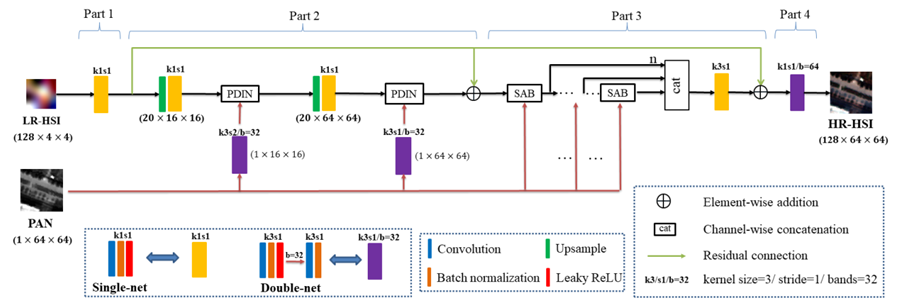Part 1为编码部分，即将输入高光谱影像投影到低维丰度空间中，采用了Conv+BN+LReLU的网络结构进行投影。

Part 2为上采样部分，由于本文关注于大比例融合任务，故采用了两步上采样来逐渐提升影像分辨率，绿色方块为上采样block，包含bicubic内插和单卷积操作。紫色方块Double-net为双卷积操作，用于匹配丰度的分辨率和提取特征。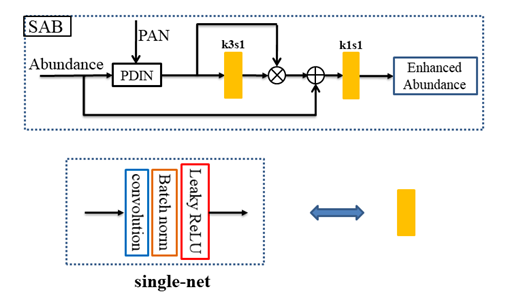Part 3为注意力增强部分，采用了联级的像素级注意力机制来进一步增强特征，如图5所示。并且SAB block中加入了PDIN来进一步注入全色空间细节。

Part 4为解码部分，即将丰度特征还原为高光谱影像。值得注意的是，这部分的卷积参数可以看成线性混合模型中的端元。

1def generate_data(path, path_srf):    # 第一步
2    ratio_hs = 16
3    # spectral response function of worldview2
4    srf_pan = np.expand_dims(np.expand_dims(np.load(path_srf), axis=-1), axis=-1)
5
6    noise_mean = 0.0
7    noise_var = 0.0001
8    dowmsample16 = Downsampler(ratio_hs)    # 高斯核降采样
10    band, row, col = original_msi.shape
11original_msi = original_msi[:, :(row - row%(ratio_hs*4)), :(col - col%(ratio_hs*4))]
12
13    # ratio 16
14    temp_blur = dowmsample16(paddle.to_tensor(np.expand_dims(original_msi, axis=0), dtype='float32'))
15    print(temp_blur.shape)
16    temp_blur = np.squeeze(temp_blur.numpy())
17    _, rows, cols = temp_blur.shape
18    blur_data = []
19    for i3 in range(temp_blur.shape):    # 加入噪声
20        blur_data.append(np.expand_dims(temp_blur[i3, :, :] +
21                                        np.random.normal(noise_mean, noise_var ** 0.5, [rows, cols]), axis=0))
22    blur_data = np.concatenate(blur_data, axis=0)
23    print('blur_data.shape:' + str(blur_data.shape))
24
25    # simulated pan image
26    temp_pan = np.expand_dims(np.sum(original_msi * srf_pan, axis=0) / np.sum(srf_pan), axis=0)
27    print('temp_pan.shape:' + str(temp_pan.shape))
28
29    return original_msi, temp_blur, temp_pan
30
31def crop_data(hrhs, lrhs, pan, ratio=16, train_ratio=0.8):        # 第二步
32    training_size = 64  # training patch size
33    testing_size = 256  # testing patch size
34    idx = int(lrhs.shape * train_ratio)    # 切割的坐标索引值
35
36    '''产生训练和测试数据'''
37    train_hs_image, train_hrpan_image, train_label = \
38        Crop_traindata(lrhs[:, :, :idx],
39                        pan[:, :, :idx*ratio],
40                        hrhs[:, :, :idx*ratio],
41                        ratio=ratio, size=training_size,
42                        test=False)
43    test_hs_image, test_hrpan_image, test_label = \
44        Crop_traindata(lrhs[:, :, idx:],
45                        pan[:, :, idx*ratio:],
46                        hrhs[:, :, idx*ratio:],
47                        ratio=ratio, size=testing_size,
48                        test=True)
49    return train_hs_image, train_hrpan_image, train_label, test_hs_image, test_hrpan_image, test_label

1#  定义数据和模型
2dataset0 = Mydata(train_hs_image, train_hrpan_image, train_label)        #  训练数据
4                                 shuffle=True, drop_last=True)
5
6dataset1 = Mydata(test_hs_image, test_hrpan_image, test_label)        #  测试数据
8                       shuffle=False, drop_last=False)
9
10model = Pg_net(band=opt.in_nc, endmember_num=opt.endmember, ratio=ratio)
11
12L2_loss = nn.loss.MSELoss()        #  loss 函数
13samloss = SAMLoss()
14
15scheduler = optim.lr.StepDecay(opt.lr, opt.step, gamma=opt.momentum, verbose=False)    #  学习率调整
17for epoch in range(opt.num_epochs):
18    time0 = time.time()
19    loss_total = 0.0
20
21    scheduler.step(epoch)
22    model.train()
23    for i, (images_hs, images_pan, labels) in enumerate(train_loader()):
24        result = model(images_hs, images_pan)
25
26    loss_l2 = L2_loss(result, labels)
27        loss_sam = samloss(result, labels)
28        loss = loss_l2 + 0.01*loss_sam
29
30        loss.backward()
31        optimizer.step()
33        loss_total += loss.numpy()
34
35    if ((epoch+1) % 10) == 0:
36        print('epoch %d of %d, using time: %.2f , loss of train: %.4f' %
37            (epoch + 1, opt.num_epochs, time.time() - time0, loss_total))
38
39# 测试结果输出：
40model.eval()
41image_all = []
43    for (images_hs, images_pan, _) in test_loader:
44        outputs_temp = model(images_hs, images_pan)
45        image_all.append(outputs_temp)
47return a

JiaXing 实验结果：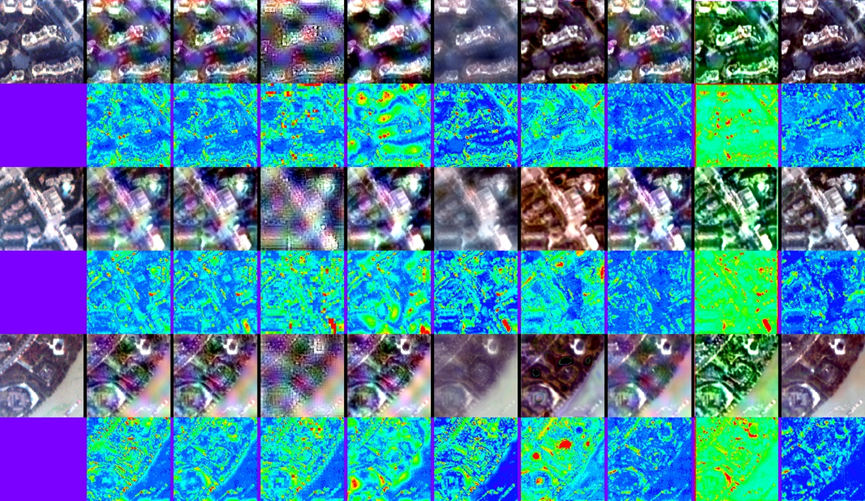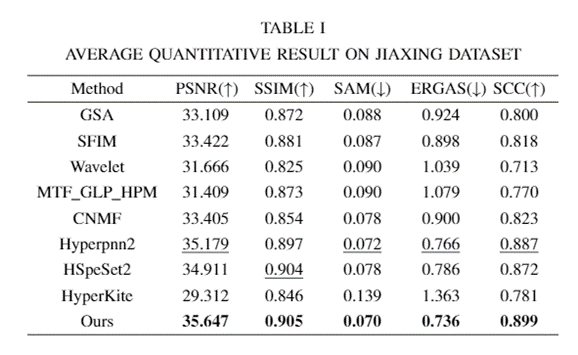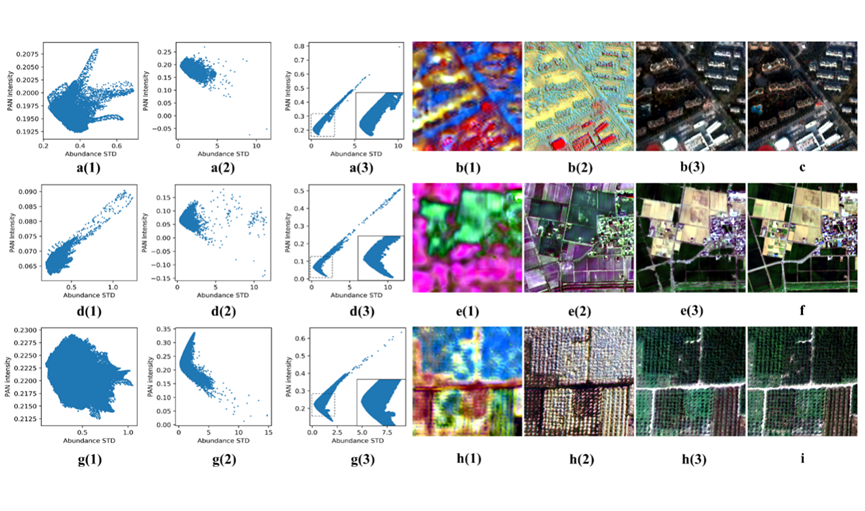🔽项目链接

https://aistudio.baidu.com/aistudio/projectdetail/3383847

🔽论文链接

https://ieeexplore.ieee.org/document/9676675

• # 美景本天成，妙笔偶得之——“妙笔”是怎样炼成的？### 作者的其它热门文章

0
1 收藏

0 评论
1 收藏
0